# Acceleration - math word problems

#### Number of problems found: 53

• Car overtaking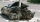A passenger car travels at a speed of 30 m/s, and before it travels freight truck that drives at a constant speed of 10 m/s. Within 30 meters of the truck, the driver of the car finds out that the truck can not overtake. That's why it starts braking with
• Bisectors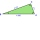As shown, in △ ABC, ∠C = 90°, AD bisects ∠BAC, DE⊥AB to E, BE = 2, BC = 6. Find the perimeter of triangle △ BDE.
• Rocket start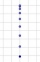The body launched vertically up returns to the start site in 6 seconds. What height did it have?
• Free fall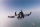How long does the stone fall freely into a depth of 80m? What speed will it hit the bottom of the abyss?
• Free fallThe free fall body has gone 10m in the last 0.5s. Find the body speed at the moment of impact.
• Fall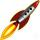The body was thrown vertically upward at speed v0 = 79 m/s. Body height versus time describe equation ?. What is the maximum height body reach?
• Free fall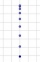For how long and at what speed does the body fall to the ground during a free fall from a height of 35 m?
• Buoyancy forceAn adult male has a volume of about 0.070 m3. How much buoyancy does it affect him when he is completely immersed in water?
• Train 2The train slowed down from 90 km/h to 72 km/h in 5 seconds. How long track travel?
• Shot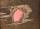Shot with a mass 43 g flying at 256 m/s penetrates into the wood to a depth 25 cm. What is the average force of resistance of wood?
• The projectileThe projectile was fired horizontally from a height of h = 25 meters above the ground at a speed of v0 = 250 m/s. Find the range and flight time of the projectile.
• Skid friction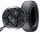Find the smallest coefficient of skid friction between the car tires and the road so that the car can drive at a 200 m radius at 108 km/h and does not skid.
• CarAt what horizontal distance reaches the car weight m = 753 kg speed v = 74 km/h when the car engine develops a tensile force F = 3061 N. (Neglect resistance of the environment.)
• Brakes of a carFor the brakes of a passenger car to be effective, it is prescribed that a car moving on a horizontal road at a speed of 40 km. A car must stop on the track 15.4 m. What is the deceleration of the car?
• Ball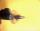The Ball was fired at an angle of 35° at an initial velocity of 292 m/s. Determine the length of the litter. (g = 9.81 m/s2).
• BomberThe aircraft flies at an altitude of 4100 m above the ground at speed 777 km/h. At what horizontal distance from the point B should be release any body from the aircraft body to fall into point B? (g = 9.81 m/s2)
• Hydrostatic forceWhat hydrostatic force is applied to an area of 30 cm2 in water at a depth of 20 m? (Water density is 1000 kg/m3)
• Up and down motionWe throw the body from a height h = 5 m above the Earth vertically upwards v0 = 10 m/s. How long before we have to let the second body fall freely from the same height to hit the Earth at the same time?
• Deceleration of carThe car has a speed of 60 km/h and after 100 m journey speed of 40 km/h. What is the deceleration of a car if we assume that its movement is constantly slowed down?
• GravitationFrom the top of the 80m high tower, the body is thrown horizontally with an initial speed of 15 m/s. At what time and at what distance from the foot of the tower does the body hit the horizontal surface of the Earth? (use g = 10 ms-2)

Do you have an interesting mathematical word problem that you can't solve it? Submit a math problem, and we can try to solve it.

We will send a solution to your e-mail address. Solved examples are also published here. Please enter the e-mail correctly and check whether you don't have a full mailbox.

Please do not submit problems from current active competitions such as Mathematical Olympiad, correspondence seminars etc...